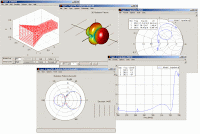## Saturday, March 8, 2008

### Parameters AntennaParameters of the antenna used to describe a performance antenna. Parameters of the antenna can be related to each other when someone wants to design an antenna, although not all parameters must be considered in depth. Type of antenna parameters include:

Radiation pattern is a graphical representation of electricity and magnetic field in the space coordinates. In many cases, the pattern radiation specified in the region and far-field is represented as a function of directional coordinates.

• Isotropic
Antenna radiation pattern ideal lossless or radiation that direction is the same to all (shaped like a ball). Radiation patterns such as this are usually used as a reference antenna with the unit of measurement dBi.

• Directional
Radiation pattern that is better for a particular direction (for unidirectional and bidirectional one direction to two directions).

• Omnidirectional
The directional radiation pattern, but all the same to the direction (the vertical axis there is no antenna radiation). Radiation pattern is shaped like a sinker. Radiation pattern like this used to broadcast communications.

Parameters comparison shows that the antenna power by the radiation emanating from an antenna per unit solid angle.

3. Directional
Parameters that indicate the value gain directive on the maximum. In a certain direction, the gain directivity comparison radiation intensity in the direction compared with the intensity radiation antenna references.

4. Gain
Parameters which indicate the ratio of power on the antenna of the antenna with a power reference. Relations between the directivity and gain can be written as follows: G = μD, where the G (Gain), D (Directivity) and μ (the ratio of the intensity of radiation).

5. Antenna efficiency
Consider the efficiency of the antenna total loss that occurs in the input terminals and an antenna inside the structure loss like this can happen because:
• reflection result does not match the transmission channel and antenna.
• Loss conduction and dielectric.

6. Half Power Beam Width (HPBW)
HPBW is the angle between two directions of radiation from half the intensity of the beam maximum value. If converted in dB, the value of HPBW obtained when the power down 3dB.

7. Efficiency beam
This parameter is often used to determine the quality of transmitting and receiving antenna. Efficiency is the ratio of beam power that emanated (received) in the cone angle compared with the power that emanated (accepted) by the antenna.

8. Bandwidth
Bandwidth is the frequency range on both sides of the center frequency (resonance frequency of the dipole antenna), which characteristics of the antenna (impedance input, the radiation pattern, beam width, polarization, the side lobe level, gain, beam direction, the efficiency of radiation) is in the optimal value. Narrow band to this antenna. Meanwhile, for Broadband, the bandwidth is a comparison between the frequency up and down in frequency levels that can be accepted.

9. Polarization
Parameters which indicate that the wave dielectric radiation when the antenna work. When the direction of polarization is not mentioned, polarization in direction is considered the maximum gain. Kind of polarization:

• linear polarization
A wave of Harmonic linear polarization at a point if the electric field (magnetic fields) in the direction of the move in a straight direction all the time.

• circular polarization
A wave of harmonic polarization circle on a point if the electric field (magnetic fields) in the direction of the move in the direction of the coil as a function of time.

• ellipse polarization
A wave of harmonic polarization linear at a point if the electric field (magnetic fields) in the direction of the move formed Locus ellipse in the space coordinates.

10. Impedance Input
This parameter shows the ratio of voltage to the antenna terminal on the flow rate or electric field component of the magnetic fields on a point or value impedance existing antenna on it.